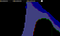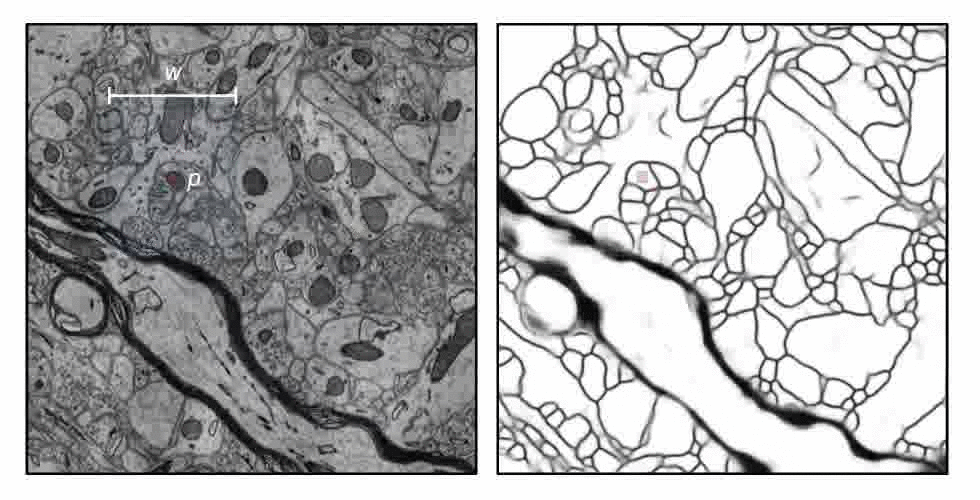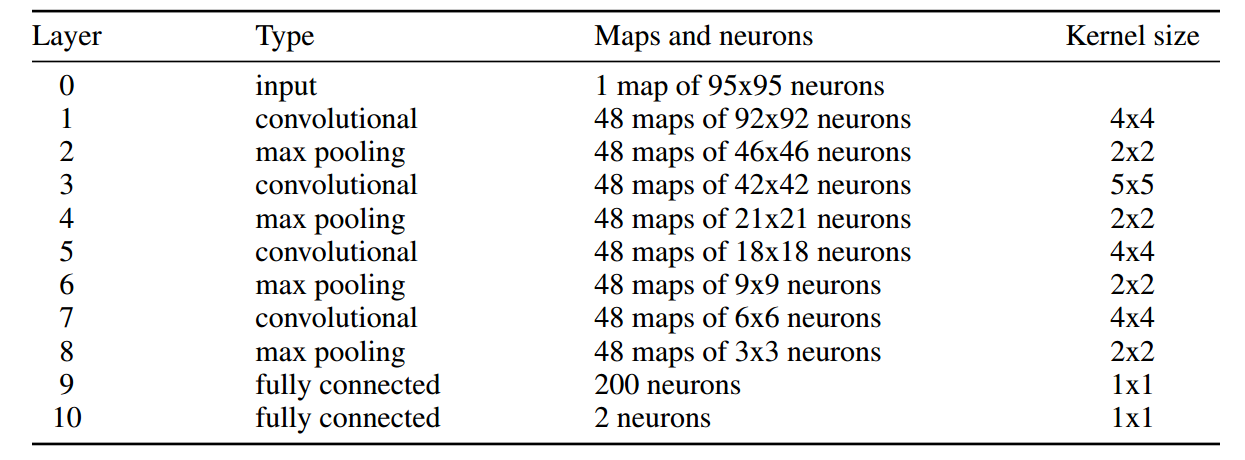# Map your brain with Deep Learning

Here is a practical guide on how to map your brain — make sure to read until the end before you start.

The first step is to cut your brain into very thin slices and take a picture of each of them. How thin? At least 40 nanometers thin.

The next step is to identify each neuron within the slices with a unique color. By doing that, we can see how these neurons are connected to each other (really useful if you are trying to stimulate your brain in a computer).

Given that you have 80 billion neurons, you might want to automate this process. Let me show you how.

In the case you want to experiment with someone else’s brain first you can download images from this challenge. Or clone this repo, which comes with some other convenient stuff.

`git clone -depth=50 https://github.com/tartavull/trace.git cd tracegit submodule update --init --recursivepip install -r requirements.txt make submodulescd tracepython cli.py download`

• train-input.h5 : a 3d array containing the electron microscopy images.
• train-labels.h5: the corresponding 3d array containing an unique number of each neurite.
• test-input.h5: another set of 3d electron microscopy images.

Why don’t we take a look at the data first?

`python cli.py visualize train`

Training a network to output a unique number for each neuron is hard, so we will transform the labels into an affinity representation. For any two consecutive pixels in the “x” dimension, if they belong to the same neuron, their affinity is 1; if they belong to different neurons, their affinity is 0. If both pixels belong to the boundary, the convention is to set the affinity as 0. We will do this for the three axes.

It is also possible to visualize all three (x,y,z) affinities simultaneously, by making x: red, y:green, and z:blue(we will ignore the z affinities for now, to only training a 2d convet).

`python cli.py visualize train --aff`By hovering over a layers in the top left is possible to change the opacity.

Time to train a neural network to predict the affinities from the input image. We will use a sliding window convnet from this paper called N4. An sliding window convnet gets a patch of input image and has two numerical outputs which corresponds to the x,y affinities of the pixel in the center of the patch.Sliding window convents predicts one point at each iteration. Animation by Kisuk Lee.Description of the convnet architecture of N4. Table from Ciresan et al. 2012.

We can write that architecture in tensorflow with the following code.

`# layer 0 image = tf.placeholder(tf.float32, shape=[None, 95, 95, 1]) target = tf.placeholder(tf.float32, shape=[None, 2])# layer 1 W_conv1 = weight_variable([4, 4, 1, 48]) b_conv1 = bias_variable() h_conv1 = tf.nn.relu(conv2d(image, W_conv1) + b_conv1)# layer 2 h_pool1 = max_pool_2x2(h_conv1)# layer 3 W_conv2 = weight_variable([5, 5, 48, 48]) b_conv2 = bias_variable() h_conv2 = tf.nn.relu(conv2d(h_pool1, W_conv2) + b_conv2)# layer 4 h_pool2 = max_pool_2x2(h_conv2)# layer 5 W_conv3 = weight_variable([4, 4, 48, 48]) b_conv3 = bias_variable() h_conv3 = tf.nn.relu(conv2d(h_pool2, W_conv3) + b_conv3)# layer 6 h_pool3 = max_pool_2x2(h_conv3)# layer 7 W_conv4 = weight_variable([4, 4, 48, 48]) b_conv4 = bias_variable() h_conv4 = tf.nn.relu(conv2d(h_pool3, W_conv4) + b_conv4)# layer 6 h_pool4 = max_pool_2x2(h_conv4)# layer 9 W_fc1 = weight_variable([3 * 3 * 48, 200]) b_fc1 = bias_variable()h_pool4_flat = tf.reshape(h_pool4, [-1, 3*3*48]) h_fc1 = tf.nn.relu(tf.matmul(h_pool4_flat, W_fc1) + b_fc1)# layer 10 W_fc2 = weight_variable([200, 2]) b_fc2 = bias_variable() prediction = tf.matmul(h_fc1, W_fc2) + b_fc2`

You can start training the network by running:

`python cli.py train`

To track the progress of training in your browser, launch tensorboard:

`tensorboard --logdir=/tmp/snemi3d/`

Once we think the network has learned the task you can create affinites for the test set with:

`python cli.py predict`

To produce labels from the predicted affinities we will run watershed.
The repo includes an implementation of watershed in the julia programming language. Here is how to install julia.

`python cli.py watershed test`

You can now visualize the the results of your training by running:

`python cli.py visualize test`